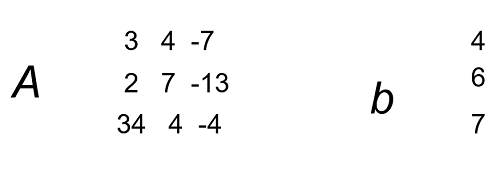# Representation of a System of Linear Equation | Linear Algebra using Python

Linear Algebra using Python | Representation of a System of Linear Equation: Here, we are going to learn about representation of a system of linear equation in Python.
Submitted by Anuj Singh, on May 23, 2020

Prerequisites:

In this article, we are going to learn how to represent a linear equation in Python using Linear Algebra. For example we are considering an equation with 3 variables (x,y,z and t).

```    3x + 4y - 7z + 12t = 46
2x + 7y - 13z + 3t = 65
34x + 4y - 4z + 34t = 78
```

The above equation has a form as below in linear Algebra:

```    Ax = b, x = (x y z t)
```Application:

1. Machine Learning
2. Calculus
3. Linear Programming
4. Physics and Kinetic Studies

## Python code for Representation of a system of linear equation

```# Linear Algebra Learning Sequence
# Representation of a System of Linear Equation

import numpy as np

# Use of np.array() to define an Vector
A = np.array([[3, 4, -7, 12], [2, 7, -13, 3], [34, 4, -4, 34]])
b = np.array([46, 65, 78])
print("The Matrix A : \n",A)

x = np.array(['x', 'y', 'z', 't'])
print("\nThe Vector x : ",x)
print("\nThe Vector b : ",b)

print("\n---Now the equations is represented in form of vector: Ax = b---")
print("This is just a python intrepetation of understanding a linear equation")
```

Output:

```The Matrix A :
[[  3   4  -7  12]
[  2   7 -13   3]
[ 34   4  -4  34]]

The Vector x :  ['x' 'y' 'z' 't']

The Vector b :  [46 65 78]

---Now the equations is represented in form of vector: Ax = b---
This is just a python intrepetation of understanding a linear equation
```

Preparation

What's New

Top Interview Coding Problems/Challenges!